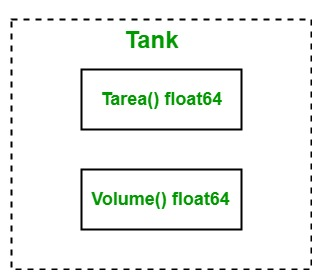GeeksforGeeks App
Open AppBrowser
Continue

Go language interfaces are different from other languages. In Go language, the interface is a custom type that is used to specify a set of one or more method signatures and the interface is abstract, so you are not allowed to create an instance of the interface. But you are allowed to create a variable of an interface type and this variable can be assigned with a concrete type value that has the methods the interface requires. Or in other words, the interface is a collection of methods as well as it is a custom type.

#### How to create an interface?

In Go language, you can create an interface using the following syntax:

```type interface_name interface{

// Method signatures

}
```

For Example:

```// Creating an interface
type myinterface interface{

// Methods
fun1() int
fun2() float64
}
```

Here the interface name is enclosed between the type and interface keywords and the method signatures enclosed in between the curly braces.

#### How to implement interfaces?

In the Go language, it is necessary to implement all the methods declared in the interface for implementing an interface. The go language interfaces are implemented implicitly. And it does not contain any specific keyword to implement an interface just like other languages. As shown in the below example:Example:

 `// Golang program illustrates how``// to implement an interface``package main`` ` `import ``"fmt"`` ` `// Creating an interface``type tank interface {`` ` `    ``// Methods``    ``Tarea() float64``    ``Volume() float64``}`` ` `type myvalue ``struct` `{``    ``radius float64``    ``height float64``}`` ` `// Implementing methods of``// the tank interface``func (m myvalue) Tarea() float64 {`` ` `    ``return` `2*m.radius*m.height +``        ``2*3.14*m.radius*m.radius``}`` ` `func (m myvalue) Volume() float64 {`` ` `    ``return` `3.14 * m.radius * m.radius * m.height``}`` ` `// Main Method``func main() {`` ` `    ``// Accessing elements of``    ``// the tank interface``    ``var t tank``    ``t = myvalue{10, 14}``    ``fmt.Println(``"Area of tank :"``, t.Tarea())``    ``fmt.Println(``"Volume of tank:"``, t.Volume())``}`

Output:

```Area of tank : 908
Volume of tank: 4396
```

#### Important Points

• The zero value of the interface is nil.
• When an interface contains zero methods, such types of interface is known as the empty interface. So, all the types implement the empty interface.

Syntax:

`interface{}`
• Interface Types: The interface is of two types one is static and another one is dynamic type. The static type is the interface itself, for example, tank in the below example. But interface does not have a static value so it always points to the dynamic values.
A variable of the interface type containing the value of the Type which implements the interface, so the value of that Type is known as dynamic value and the type is the dynamic type. It is also known as concrete value and concrete type.

Example:

 `// Go program to illustrate the concept``// of dynamic values and types``package main`` ` `import ``"fmt"`` ` `// Creating an interface``type tank interface {`` ` `    ``// Methods``    ``Tarea() float64``    ``Volume() float64``}`` ` `func main() {`` ` `    ``var t tank``    ``fmt.Println(``"Value of the tank interface is: "``, t)``    ``fmt.Printf(``"Type of the tank interface is: %T "``, t)``}`

Output:

```Value of the tank interface is:  <nil>
Type of the tank interface is: <nil>
```

Here, in the above example, we have an interface named as a tank. In this example,
fmt.Println(“Value of the tank interface is: “, t) statement returns the dynamic value of the interface and fmt.Printf(“Type of the tank interface is: %T “, t) statement returns the dynamic type, i.e nil because here the interface does not know who is implementing it.

• Type Assertions: In Go language, type assertion is an operation applied to the value of the interface. Or in other words, type assertion is a process to extract the values of the interface.

Syntax:

`a.(T)`

Here, a is the value or the expression of the interface and T is the type also known as asserted type. The type assertion is used to check that the dynamic type of its operand will match the asserted type or not. If the T is of concrete type, then the type assertion checks the given dynamic type of a is equal to the T, here if the checking proceeds successfully, then the type assertion returns the dynamic value of a. Or if the checking fails, then the operation will panics. If the T is of an interface type, then the type assertion checks the given dynamic type of a satisfies T, here if the checking proceeds successfully, then the dynamic value is not extracted.

Example:

 `// Go program to illustrate``// the type assertion``package main`` ` `import ``"fmt"`` ` `func myfun(a interface{}) {`` ` `    ``// Extracting the value of a``    ``val := a.(string)``    ``fmt.Println(``"Value: "``, val)``}``func main() {`` ` `    ``var val interface {``    ``} = ``"GeeksforGeeks"``     ` `    ``myfun(val)``}`

Output:

`Value:  GeeksforGeeks`

In the above example if we change this val := a.(string) statement into val := a.(int), then the program panic. So to overcome this problem we use the following syntax:

`value, ok := a.(T)`

Here if the type of the a is equal to T, then the value contains the dynamic value of the a and ok will set to true. And if the type of the a is not equal to T, then ok set to false and value contain zero value, and the program does not panic. As shown in the below program:

Example:

 `// Go program to illustrate type assertion``package main`` ` `import ``"fmt"`` ` `func myfun(a interface{}) {``    ``value, ok := a.(float64)``    ``fmt.Println(value, ok)``}``func main() {`` ` `    ``var a1 interface {``    ``} = 98.09`` ` `    ``myfun(a1)`` ` `    ``var a2 interface {``    ``} = ``"GeeksforGeeks"`` ` `    ``myfun(a2)``}`

Output:

```98.09 true
0 false
```
• Type Switch: In Go interface, type switch is used to compare the concrete type of an interface with the multiple types provide in the case statements. It is similar to type assertion with only one difference, i.e, case specifies types, not the values. You can also compare a type to the interface type. As shown in the below example:

Example:

 `// Go program to illustrate type switch``package main`` ` `import ``"fmt"`` ` `func myfun(a interface{}) {`` ` `    ``// Using type switch``    ``switch` `a.(type) {`` ` `    ``case` `int``:``        ``fmt.Println(``"Type: int, Value:"``, a.(``int``))``    ``case` `string:``        ``fmt.Println(``"\nType: string, Value: "``, a.(string))``    ``case` `float64:``        ``fmt.Println(``"\nType: float64, Value: "``, a.(float64))``    ``default``:``        ``fmt.Println(``"\nType not found"``)``    ``}``}`` ` `// Main method``func main() {`` ` `    ``myfun(``"GeeksforGeeks"``)``    ``myfun(67.9)``    ``myfun(``true``)``}`

Output:

```Type: string, Value:  GeeksforGeeks

Type: float64, Value:  67.9